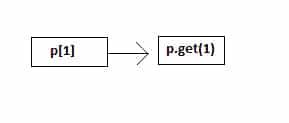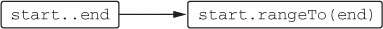We all know, `Kotlin` use the principle of a convention, instead of relying on types of  `Java` does, because this allows developers to adapt existing `Java` classes to the requirement of `Kotlin` language features. The set of interfaces implemented by a class is fixed, and `Kotlin` can’t modify an existing class so that it would implement additional interfaces.

#### What is Kotlin Conventions:

For Example, if your class defines a special method name `plus` , then, by convention you can use the `+` operator on the instance of this class. Because of that `Kotlin` refers to this technique as Kotlin Conventions. Similarly, the same technique applies on get, set, in, and on a rangeTo convention.

So, enough of this theory let’s see the example first of how we can use these conventions in our application.

##### 1. Accessing Element By get Convention

You guy’s, already know that in `Kotlin` , you can access the elements in a `Map` similarly to how you access `Arrays` in java–via square brackets:

`val value = map[key]`

Meanwhile, it’s time to see how this works. In `Kotlin` , the index operator is one more convention. Reading the element using the index operator is translated into a call of `get` operator method. Generally, the method is already defined for the `Map` and `MutableMap` interfaces. Let’s see how to add a similar method for your own class:

```fun main(args: Array<String>) {

val p = Point(10, 20)
val second = p
println(second)
}

data class Point(val x: Int, val y: Int)

operator fun Point.get(index: Int): Int {
return when (index) {
0 -> x
1 -> y
else ->
throw IndexOutOfBoundsException("Invalid coordinate \$index")
}
}

// Output of above program
20```

You see we use the square brackets reference the coordinates of the point. Similarly, all you need to do is to define a function name `get` and mark it as `operator`. Once you do that, the expression like `p`, where p has type `Point`, will be translated into calls to the `get` method.Note: The parameter `get` can be any type, not just `Int`. For Example, when you indexing operator on a `Map`, the parameter type is the key type of the `Map`, which can be an arbitrary type. You can define multiple overloaded `get` methods with different parameter types if your collection can be accessed with different key types.

##### 2. Assign Element By set Convention

The same goes for the `set` convention that lets you to change the value at a given index using a bracket index. Let’s define a mutable `Point` class and use that an example:

```fun main(args: Array<String>) {
val p = Point(10, 20)
p = 35
}

data class Point(var x: Int, var y: Int)

operator fun Point.set(index: Int, value: Int) {
when (index) {
0 -> x = value
1 -> y = value
else ->
throw IndexOutOfBoundsException("Invalid coordinate \$index")
}
}```

To use the index operator in the assignment, you just need to define a function name `set`. The last parameter to `set` receives the value used on the right side of the assignment and the other arguments are taken from the indices used inside the brackets.

One other operator supported by collections is the `in` operator, which is used to check whether an object belongs to a collection. The corresponding function is called `contains`. Let’s implement it so that you can use the `in` operator to check whether a point belongs to a rectangle.

```data class Point(val x: Int, val y: Int)

data class Rectangle(val upperLeft: Point, val lowerRight: Point)

operator fun Rectangle.contains(p: Point): Boolean {
return p.x in upperLeft.x until lowerRight.x &&
p.y in upperLeft.y until lowerRight.y
}

fun main(args: Array<String>) {
val firstPoint = Point(10, 20)
val secondPoint = Point(50, 50)
val rect = Rectangle(firstPoint, secondPoint)
println(Point(15, 20) in rect)
println(Point(8, 8) in rect)
}

// Output of above program

true
false```

The object on the right side of `in` becomes the object on which the `contains` method is called and the object on the left side becomes the argument passed to the method. Furthermore, in the implementation of `Rectangle.contains` , you see we use the `until` standard library function to build an open range and then you use the `in` operator on a range to check that a point belongs to it or not.

To create a range, you use the `..` syntax: for-instance,  `1..10` enumerate all the numbers from 1 to 10. The `..` operator under the hood call the `rangeTo` method:The `rangeTo` function defines a range. Moreover, you can define this operator for your own class. But if your class define the `Comparable` interface, you don’t need that: you can create a range of any comparable elements by means of the Kotlin standard library.

Note: The library defines the `rangeTo`  function that can be called on any comparable element:

`operator fun <T: Comparable<T>> T.rangeTo(that: T): ClosedRange<T>`

This function returns a range that allows you to check whether different elements belong to it.

As an example, let’s see build a range of points using `Point` class.

```data class Point(val x: Int, val y: Int) : Comparable<Point> {
override fun compareTo(other: Point) = (this.x + y).compareTo(other.x + other.y)
}

fun main(args: Array<String>) {
val ranges = Point(20, 20)..Point(20, 40)
val firstResult = Point(25, 20) in ranges
val secondResult = Point(10, 10) in ranges
println(firstResult)
println(secondResult)
}

// The output of above program :
true
false```

The expression `Point(20,20)..Point(20,40)` is transformed into  `Point(20,20).rangeTo(Potin(20,40))` by the compiler. The `rangeTo` isn’t a member of `Point` class but rather is an extension function on `Comparable`, as shown earlier. The  `in` operator in above program only return `true`, if the `Point` x and y coordinate value is in the range `40-60`.

By defining the function named `get`,  `set` , and  `contains`, you can support the  `[]` and  `in` operators to make your class similar to  `Kotlin` collections.

That’s it. As a whole, this is my knowledge about Kotlin Conventions. I tried to learn from my mistakes, others’ and my experiences. I’ve been constantly finding the best approach.

I hope you liked this article. Besides this, if you have any feedback, feel free to comment below: I would be very happy to get suggestions.

Thank you for being here and keep reading…I’m a mobile product devsigner (i.e. I consider myself as both a developer and a designer) and user experience/interface engineer. I’m an expert on the Android platform and have been recognized as it by the community.